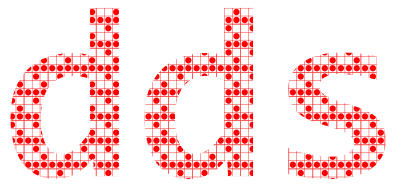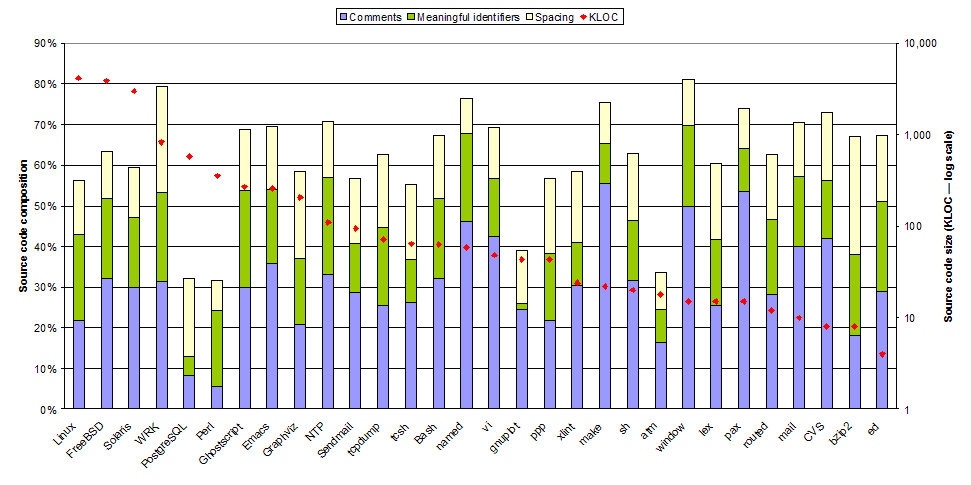Diomidis Spinellis blog## The Way We Program

If the code and the comments disagree, then both are probably wrong.

— Norm Schryer

I can still remember the first time I laid my eyes on production-quality source code. This was in the early 1980s and the code was the BIOS listing of the original IBM PC. The 5940 lines of code spanned 80 neatly typeset pages in a three-ring slip-covered binder. Two things made a lasting impression on me. The first was the elation of being able to read, understand and learn from the code that made a real machine tick. This may have sparked my current practical and research interests in open source software. The second, was the way the code was commented. The BIOS was written in 8086 assembly language, and almost every line had a comment on its right hand side. By poring over the code and its comments I learned 8086 assembly, programming style, and understood the PC’s hardware architecture.

 ```#define O(b,f,u,s,c,a)b(){int o=f();switch(*p++){X u:_ o s b();X c:_ o a b();default:p--;_ o;}} #define t(e,d,_,C)X e:f=fopen(B+d,_);C;fclose(f) #define U(y,z)while(p=Q(s,y))*p++=z,*p=' ' #define N for(i=0;i<11*R;i++)m[i]&& #define I "%d %s\n",i,m[i] #define X ;break;case #define _ return #define R 999 typedef char*A;int*C,E[R],L[R],M[R],P[R],l,i,j;char B[R],F;A m[12*R],malloc (),p,q,x,y,z,s,d,f,fopen();A Q(s,o)A s,o;{for(x=s;*x;x++){for(y=x,z=o;*z&&*y== *z;y++)z++;if(z>o&&!*z)_ x;}_ 0;}main(){m[11*R]="E";while(puts("Ok"),gets(B) )switch(*B){X'R':C=E;l=1;for(i=0;i",'#');U("<=",'\$');U(">=",'!');}d=B;while(*F=*s){*s=='"'&&j ++;if(j&1||!Q(" \t",F))*d++=*s;s++;}*d--=j=0;if(B!='=')switch(*B){X'E':l=-1 X'R':B!='M'&&(l=*--C)X'I':B=='N'?gets(p=B),P[*d]=S():(*(q=Q(B,"TH"))=0,p =B+2,S()&&(p=q+4,l=S()-1))X'P':B=='"'?*d=0,puts(B+6):(p=B+5,printf("%d\n",S ()))X'G':p=B+4,B=='S'&&(*C++=l,p++),l=S()-1 X'F':*(q=Q(B,"TO"))=0;p=B+5;P[i =B]=S();p=q+2;M[i]=S();L[i]=l X'N':++P[*d]<=M[*d]&&(l=L[*d]);}else p=B+2,P[ *B]=S();l++;}X'L':N printf(I)X'N':N free(m[i]),m[i]=0 X'B':_ 0 t('S',5,"w",N fprintf(f,I))t('O',4,"r",while(fgets(B,R,f))(*Q(B,"\n")=0,G()))X 0:default:G() ;}_ 0;}G(){l=atoi(B);m[l]&&free(m[l]);(p=Q(B," "))?strcpy(m[l]=malloc(strlen(p )),p+1):(m[l]=0,0);}O(S,J,'=',==,'#',!=)O(J,K,'<',<,'>',>)O(K,V,'\$',<=,'!',>=) O(V,W,'+',+,'-',-)O(W,Y,'*',*,'/',/)Y(){int o;_*p=='-'?p++,-Y():*p>='0'&&*p<= '9'?strtol(p,&p,0):*p=='('?p++,o=S(),p++,o:P[*p++];} ```

Figure 1. A program as a code blob: a BASIC Interpreter

So how important are comments in the programs we write? To answer this question I set out to measure the percentage of source code size occupied by comments in a few programs. It then occurred to me that comments are only one of the mechanisms we developers use to communicate with our colleagues through the source code. Other mechanisms are the creation of meaningful identifiers, the laying out of the program with whitespace, the use of language-provided high-level abstractions, and the development of our own abstractions. To get a feeling of how important these mechanisms are, have a look at Figure 1 listing a BASIC interpreter implemented in 1536 bytes. I wrote that program with the express goal to communicate as little information as possible, in order to submit it to the 1990 International Obfuscated C Code Contest. The code uses single-letter identifiers, employs minimal whitespace, and doesn’t contain any pesky comments. This is the type of code that can keep us awake at night; a literal and metaphorical nightmare.Figure 2. Developer-oriented elements in the composition of source code

To see how we use these three mechanisms in practice, I took 30 programs of various sizes and measured what percentage of their source code consisted of comments and whitespace. I also calculated the percentage of source code size devoted to meaningful identifiers by counting the number of (non-library) unique identifiers, then deriving the minimum number of characters required for expressing them, and finally obtaining the corresponding source code size savings we could obtain by using the smallest possible identifier names. (It turns out that for programs up to 15 thousand lines of code (KLOC) you can get away with two-character identifiers, and for all but the three largest systems I measured—the Linux, Solaris, and FreeBSD kernels—three-character identifiers are perfectly adequate. And this calculation is conservative, because I ignored the savings one can obtain by reusing identifiers in different scopes. Malfeasant programmers and language designers take note.) You can see a graphical summary of this small study in Figure 2.

I found the results surprising: in the source code of a typical system more than 50% of the code serves not as instructions to the computer, but as a communications vehicle targeting developers. Furthermore, although we often discuss the importance of comments (this was my original idea for this column), it seems that programmers devote to meaningful identifiers and even the humble whitespace almost the same area of screen real estate. Therefore presumably, all three mechanisms are equally important. In addition, although I was expecting that the measured composition of a program’s elements would differ according to a program’s size, my data didn’t show such a variation for programs spanning a range from millions to thousands of code lines. It seems that for any non-trivial program each of these three mechanisms carries equal weight; one could well posit that this reflects a stylistic equilibrium we reached through evolution. Finally, although the ratio of code serving the developers rather than the compiler seems high, note that it doesn’t even include the overhead of code employed for building and using various abstractions, such as method definitions and calls. Although some abstractions can result in more compact code, my feeling is that on balance abstractions (deservedly) contribute positively to a system’s source code size.

### Implications

I don’t think that anybody in their right mind would ever dream of writing or even representing the Linux kernel as a blob similar to that in Figure 1 (at least not before reading this column). Nevertheless, I find the large part of the source code that is explicitly targeting developers profoundly significant, because it confirms my belief that source code is the most important artifact of the software development process. Programming is not coding. Programming is not the mechanical transfer of a software design into a form the computer can execute. As many others have observed, programming is an art, and the three elements I’ve measured are separate identifiable artistic expressions. The writing of comments is prose literature, aiming to tell the story behind the code. The formation of layout through whitespace is sculpture, seeking to show the code’s hidden structure. Finally, the choice of meaningful identifiers is almost stylized poetry: a type of creative communication through a few words adhering to a specific form. Our overarching goal is to communicate effectively: plainly, succinctly, and unambiguously.

The large amount of creativity that goes into the software source code has several practical implications. It means that we can get great code by hiring talented developers, and compensating them in the form we would pay a great artist (ideally not the archetypal starving one). It also means that we should take care of code, treating it as a prized possession. We should learn, respect, apply, and preserve style guidelines and naming conventions; we should treat each comment as part of an essay that will be marked by the most exacting English teacher we’ve ever had.

To paraphrase William Ward: The mediocre code compiles. The good code runs. The superior code passes tests and inspections. The great code inspires.

* This piece has been published in the IEEE Software magazine Tools of the Trade column, and should be cited as follows: Diomidis Spinellis. The Way We Program. IEEE Software, 25(4):89–91, July/August 2008. (doi:10.1109/MS.2008.101)